Courses

# Test: Surface Area & Volumes- 1

## 25 Questions MCQ Test Mathematics (Maths) Class 9 | Test: Surface Area & Volumes- 1

Description
This mock test of Test: Surface Area & Volumes- 1 for Class 9 helps you for every Class 9 entrance exam. This contains 25 Multiple Choice Questions for Class 9 Test: Surface Area & Volumes- 1 (mcq) to study with solutions a complete question bank. The solved questions answers in this Test: Surface Area & Volumes- 1 quiz give you a good mix of easy questions and tough questions. Class 9 students definitely take this Test: Surface Area & Volumes- 1 exercise for a better result in the exam. You can find other Test: Surface Area & Volumes- 1 extra questions, long questions & short questions for Class 9 on EduRev as well by searching above.
QUESTION: 1

Solution:
QUESTION: 2

Solution:
QUESTION: 3

### The maximum length of a pencil that can be kept in a rectangular box of dimensions 8 cm × 6 cm × 2 cm, is:

Solution:
QUESTION: 4

How many bricks, each measuring 25 cm × 12.5 cm × 7.5 cm will be needed to construct a wall 15 m long, 1.8 m high and 37.5 cm thick?

Solution: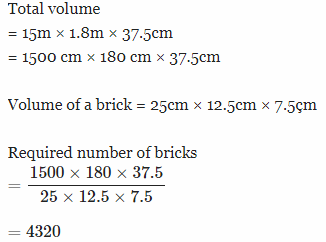QUESTION: 5

A wooden box of dimensions 8 m × 7 m × 6 m is to carry rectangular boxes of dimensions 8 cm × 7 cm × 6 cm. The maximum number of boxes that can be carried in the wooden box, is :

Solution:
QUESTION: 6

The surface area of a cube of side 27 cm is :

Solution:
QUESTION: 7

The perimeter of one face of a cube is 40 cm. The volume of the cube (in cm3) is :

Solution:
QUESTION: 8

The volume of a cube with surface area 384 sq. cm, is :

Solution:
QUESTION: 9

The length of the longest rod that can fit in a cubical vessel of side 10 cm, is

Solution:
QUESTION: 10

If the length of diagonal of a cube is 4√3  cm, then the length of its edge is :

Solution: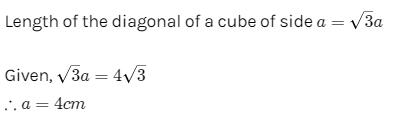QUESTION: 11

If the diameter of the base of a cylindrical pillar is 4 m and its height is 21 m, then the cost of construction of the pillar at Rs. 1.50 per cubic metre is :

Solution:
QUESTION: 12

The volume of the cylinder whose height is 14 cm and diameter of base 4 cm, is :

Solution:
QUESTION: 13

Given that 1 cm3 of a metal weighs 5 gms, the weight of a cylindrical metal container with base radius 10.5 cm and height 60 cm, is :

Solution:
QUESTION: 14

If the diameter of a cylinder is 28 cm and its height is 20 cm, then total surface area (in cm2) is :

Solution:
QUESTION: 15

If the curved surface area of a cylinder is 1760 sq. cm and its base radius is 14 cm, then its height is :

Solution:
QUESTION: 16

The volume (in cm3) of a right circular cone of height 12 cm and base radius 6 cm, is :

Solution:
QUESTION: 17

If the radius of the base of a right circular cone is 3r and its height is equal to the radius of the base, then its volume is :

Solution:
QUESTION: 18

The lateral surface area (in cm2) of a cone with height 3 cm and radius 4 cm, is :

Solution:
QUESTION: 19

A cone of height 8 m has a curved surface area 188.4 2. The radius of the base is : (Take π = 3.14) :

Solution:
QUESTION: 20

The length of canvas 1.1 m wide required to build a conical tent of height 14 m and the floor area 346.5 m2, is

Solution:
QUESTION: 21

The volume of a sphere is 38808 cu.cm. The curved surface area of the sphere (in cm2) is :

Solution:
QUESTION: 22

The volume of a spherical shell whose internal and external diameters are 8 cm and 10 cm respectively (in cubic cm) is:

Solution:
QUESTION: 23

If the ratio of volumes of two spheres is 1 : 8, then the ratio of their surface areas is :

Solution:
QUESTION: 24

If a hemi-spherical dome has an inner diameter of 28 m, then its volume (in m3) is :

Solution:
QUESTION: 25

Three solid spherical beads of radii 3 cm, 4 cm and 5 cm are melted into a spherical bead. Its radius is :

Solution:

Let three spheres are S1, S2 & S3
having radii r₁ = 3cm, r₂ = 4cm & r₃ = 5cm respectively.
Let the radius of new Big sphere S is R.
A/Q,
Volume of new Sphere  S = Sum of volumes three Spheres S1, S2 & S3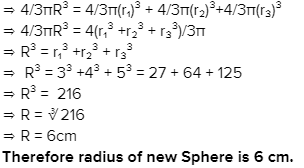Track your progress, build streaks, highlight & save important lessons and more!

### Similar Content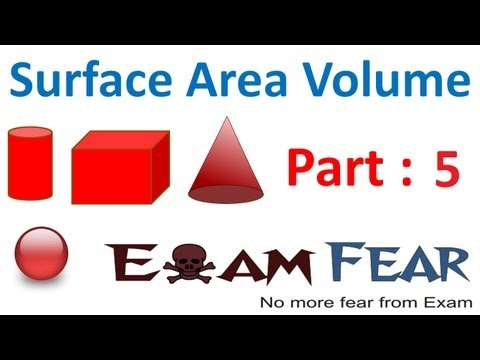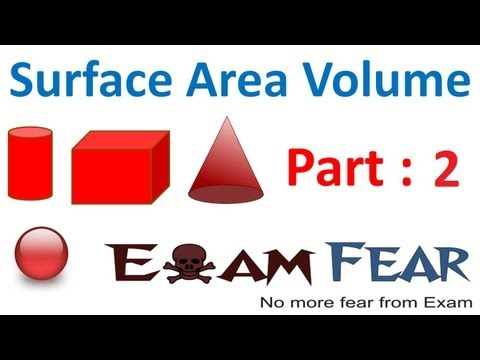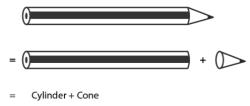### Related tests